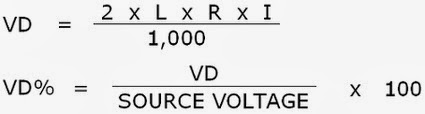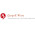## Thursday, January 16, 2014

### Episode 5 pt 3: Voltage Drop and Wire Sizing for Solar

Voltage drop and wire size are important considerations when designing and installing solar PV systems.  Voltage drop occurs in any electrical circuit carrying power. A percentage of the power running through the conductors will be lost as heat.  This amount is dependent on the size of the wire along with the voltage and current of the load, and some environmental factors as well (circuit length, temperature, conductor material).  This post will cover selecting the correct wire size based on your maximum solar current and calculating the voltage drop based on that.  I will be heavily referencing the NEC 2011 codebook throughout this post.

Conditions of Use

Before getting into selecting wire sizes, I want to cover conditions of use.  These are factors that need to be applied to the maximum current we calculated earlier based on environmental conditions.  The most obvious condition of use is ambient temperature, found in Table 310.15(B)(2)(a).  Based on most sources, the average high temperature for the location should be used.  This can be obtained from the ASHRAE handbook (the 2% value) or determined from weather data available online.  The other conditions of use are more complicated.  Conduit fill is an important consideration for large systems and long conduit runs, it is found in Table 310.15(B)(3)(a).  This is because wiring will generate heat during operation and the hotter it gets inside the conduit the worse the voltage drop will get.  Conduit distance above roof for roof mounted systems is very important as well, and can make the conductors even hotter, reference Table 310.15(B)(3)(c) for more information.  For the purpose of this post, conduit fill and conduit distance factors will both be assumed as 1 for power production.  Ambient design temperature will be fixed at 85° F, which gives a modifier of 1 on our current calculation for simplicity.

Wire Sizing

From my previous submission, maximum DC solar current is based on the solar panel’s Isc rating multiplied by 1.56.  The maximum AC solar current is the inverter’s maximum continuous current multiplied by 1.25.  Taking these maximum calculated currents, proper wire sizes for them can be selected using NEC 2011 wire sizing methods and tables.  These methods will assume conductors and terminals are rated for 75° C.  The first table to reference is 310.15(B)(16), where the 75° C Copper column will be used to select wire size based on the maximum calculated current.  For example, for a solar panel Isc rating of 8.5A, our maximum DC current is 13.26A.  Using table 310.15(B)(16), the minimum wire size #14 AWG.  For a solar inverter with a rated current of 25A, maximum AC current will be 31.25A.  This results in #8 AWG wire.  Conditions of use, if we had them, would be applied here to the overall ampacity of the wire to derate it and check against our maximum calculated current.  Conveniently, all of our conditions of use for this example are fixed at “1”.

Now that the wire size has been found for the maximum calculated current, it needs to be checked against worst case voltage drop in the conductor for the designed length of the circuit.  If the voltage drop would be too high the wire has to be upsized to the next size to correct for it.  The maximum voltage drop is 1.5% for DC and 1.5% for AC circuits based on my previous post.

Calculating Voltage Drop

The heart of voltage drop calculations is Ohm’s law, which reads V (Voltage) = I (Current) x R (Resistance). The units are Volts, Amps, and Ohms, respectively.  Voltage drop calculations are based on values for Resistance (Ohms) per 1000 feet found in NEC tables in Chapter 9, Table 8 or 9 (DC circuits Table 8,  AC circuits Table 9). The value we need is for stranded, uncoated copper conductors.  This changes the formula from V = I x R to Voltage Change = I x (R / 1000’) x Total Circuit Length
Written another way, it can be expressed in terms of 1-way circuit length and converted into a percentage value:Equation for Voltage Drop based on NEC 2011 Chaper 9 Tables 8 & 9

Going back to my example in Wire Sizing, let’s consider a DC and an AC wire run for a 100 foot distance and calculate the voltage drop for each.  For DC, we have:

#14 AWG Wire  300V String         I = 13.26A            L = 100 feet         R = 3.26 (NEC Chapter 9 Table 8)

VD = 2 x 100 x 13.26 x 3.14 / 1000 = 8.33V               8.33V / 300V = 2.78% Voltage Drop

Based on our maximum desired DC voltage drop of 1.5%, this obviously will not do.  We need to upsize the wire to get a better voltage drop.  Rerunning the numbers for a #12 wire gives:

VD = 2 x 100 x 13.26 x 1.98 / 1000 = 5.44V               5.44V/ 300V = 1.75% Voltage Drop

Close, but not quite what we wanted.  Obviously a #10 AWG wire is the way to go, but just to be sure:

VD = 2 x 100 x 13.26 x 1.24 / 1000 = 3.42V               3.42V / 300V = 1.10% Voltage Drop

That’s got it right there!  A #10 AWG wire will give us ~1.1% voltage drop for a 300V circuit over 100 feet (In general, I’ve found that a #10 wire is best for solar string wiring for most roofs).  Now that we’ve figured out DC voltage drop, AC voltage drop is pretty simple.  Using Table 9 of Chapter 9, we need to use the values in the column labeled “Alternating-Current Resistance for Uncoated Copper Wires”.  Going back to our earlier example:

#8 AWG Wire     240V AC               I = 31.25A            L = 100 feet         R = 0.78 (NEC Chapter 9 Table 9)

VD = 2 x 100 x 31.25 x .78 / 1000 = 4.875V               4.875V / 240V = 2.03% Voltage Drop

Again, we’re close but not quite where we want to be for this AC wire run.  Upsizing the wire to #6 results in:

VD = 2 x 100 x 31.25 x .49 / 1000 = 3.06V 3.06V / 240V = 1.28% Voltage Drop

Based on our examples, a #10 wire will give us a 1.1% voltage drop for a 300V, 8.5A string of solar panels over 100 feet.  A #6 wire will give us a 1.28% voltage drop for a 240V, 25A solar inverter over 100 feet.

Wire sizing and voltage drop calculations are an extremely important consideration for any solar designer or installer, and it’s essential to understand the fundamentals behind them.  The calculations and examples in this post have been kept simple on purpose, the subject can get complicated in a hurry when multiple conditions of use need to be applied.  In my next post, I will share some of my favorite tools and rules of thumb for calculating wire size and voltage drop.

References

NEC 2011
Conditions of Use: 310.15(B)(2), 310.15(B)(3)
Wire Ampacity: Table 310.15(B)(16)
Wire Resistance: Chapter 9 Tables 8 & 9

#### 1 comment:

1.Hey,
Really Nice Information!!
Now I know very relevant information about wires their resistances with formula.
Thanks for sharing:)

Regards
Ganpati Wires